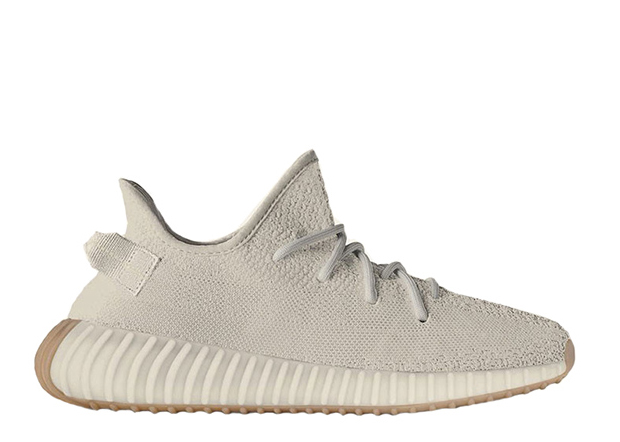All Categories
US\$

#### Cart

Home>Adidas>Yeezy Boost 350 V2>Yeezy Boost 350 V2 A1 Batch>Yeezy 350 Boost V2 Sesame (LN5 A1)

# Yeezy 350 Boost V2 Sesame (LN5 A1)

US\$ 128.00
Quality Control:
QC by myself (1-3 days prepation time) QC by FKZ team (Get shipped faster)
US10.5=UK10=EU442/3=28.5
US\$ 128.00
US10=UK9.5=EU44=28CM
US\$ 128.00
US11.5=UK11=EU46=29.5CM
US\$ 128.00
US11=UK10.5=EU451/3=29CM
US\$ 128.00
US12.5=UK12=EU47.3=30.5CM
US\$ 128.00
US12=UK11.5=EU46.7=30CM
US\$ 128.00
US4.5=UK4=EU36.7=22.5CM
US\$ 128.00
US4=UK3.5=EU36=22CM
US\$ 128.00
US5.5=EUR38=UK5=23.5CM
US\$ 128.00
US5=UK4.5=EU37.5=23CM
US\$ 128.00
US6.5=UK6=EU39=24.5CM
US\$ 128.00
US6=UK5.5=EU38.5=24CM
US\$ 128.00
US7.5=UK7=EU402/3=25.5CM
US\$ 128.00
US7=UK6.5=EU40=25CM
US\$ 128.00
US8.5=UK8=EU42=26.5CM
US\$ 128.00
US8=UK7.5=EU411/3=26CM
US\$ 128.00
US9.5=UK9=EU431/3=27.5CM
US\$ 128.00
US9=UK8.5=EU422/3=27CM
US\$ 128.00
Quantity: 0 , Subtotal 0
• Detail

Hi ,flightkickz fam, these V2 quality is good too. we dropped the price on them because they are not such popular, we need to rid them faster, please be assured to purchase them!